# RD Sharma Solutions for Class 11 Maths Exercise 24.2 Chapter 24 - The Circle

In Exercise 24.2 of Chapter 24, we shall discuss problems based on the concepts of the general equation of a circle. Students who wish to strengthen their knowledge and build a strong command over the subject can refer to RD Sharma Class 11 Maths Solutions. Experts tutors have designed the solutions in a simple and understandable language, in order to meet the requirements of students and help them score well in the board exams. Here, the solutions to this exercise are provided in pdf format, which can be downloaded easily from the links given below.

## Download the pdf of RD Sharma Solutions for Class 11 Maths Exercise 24.2 Chapter 24 – The Circle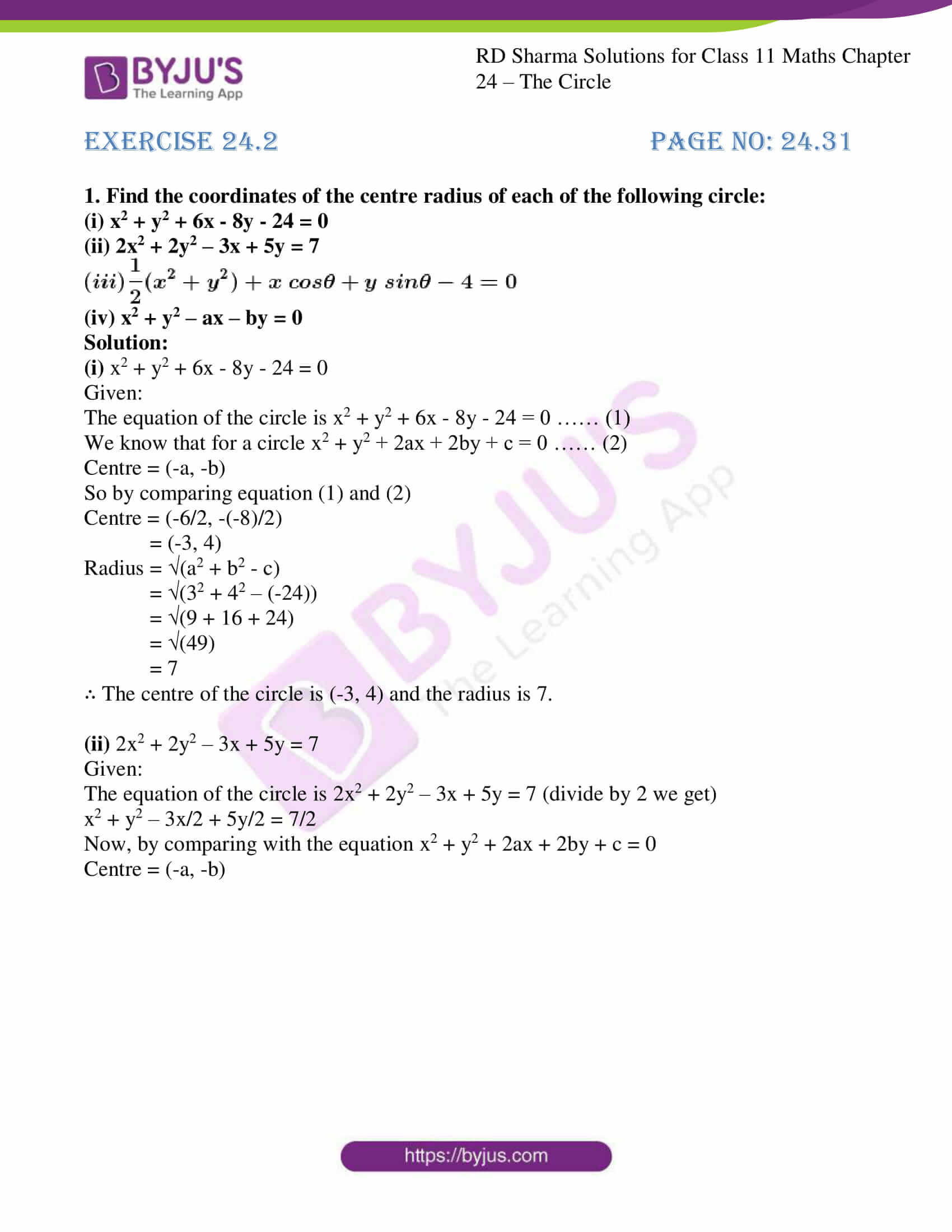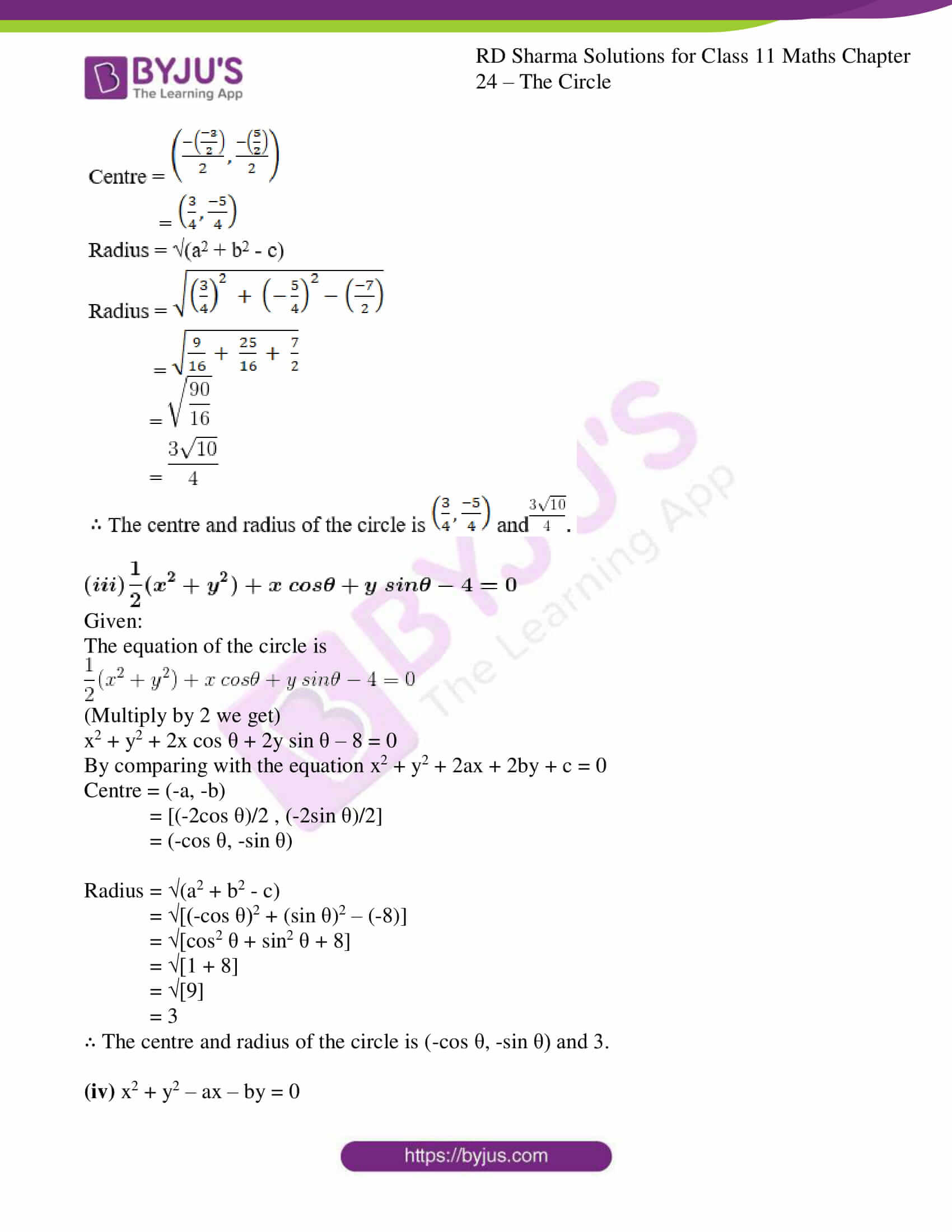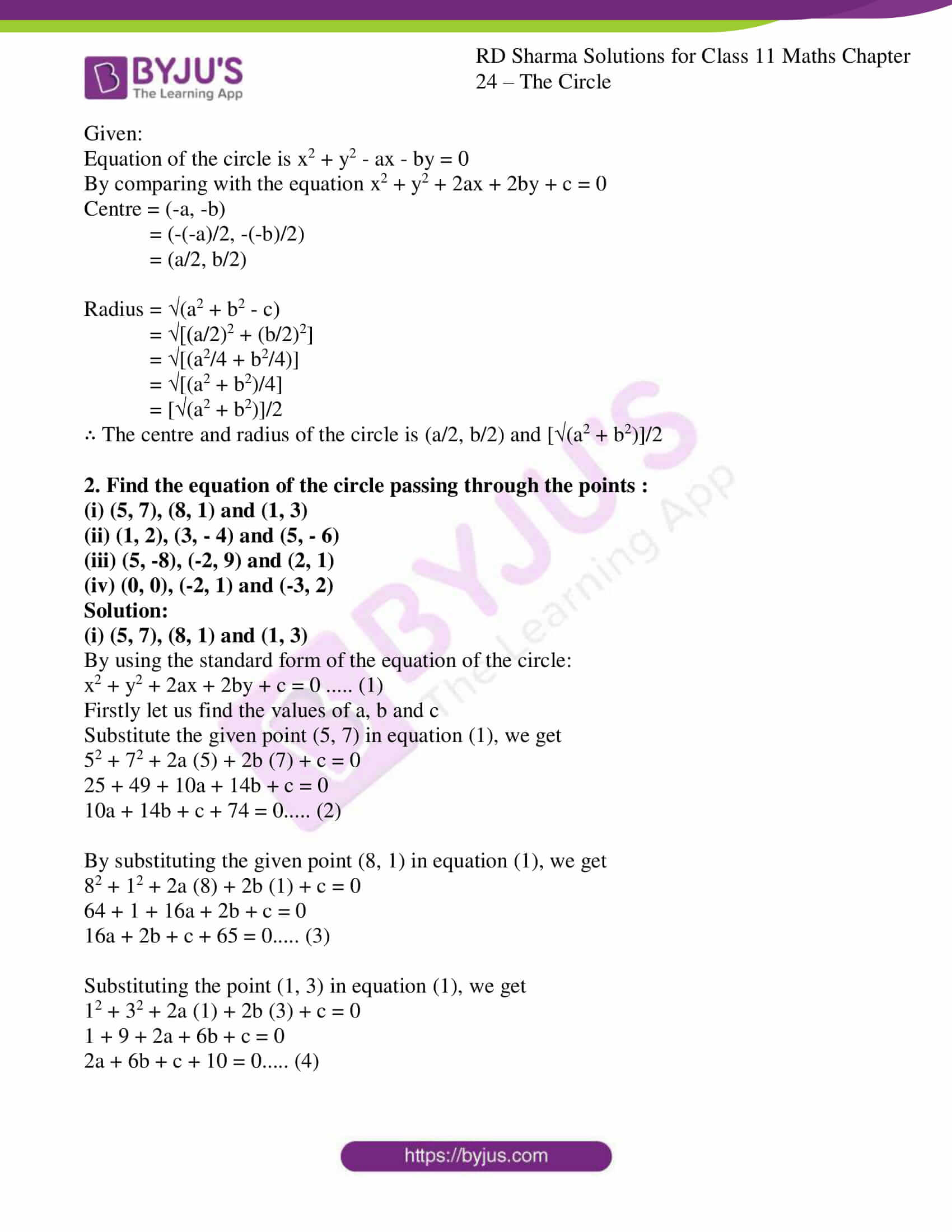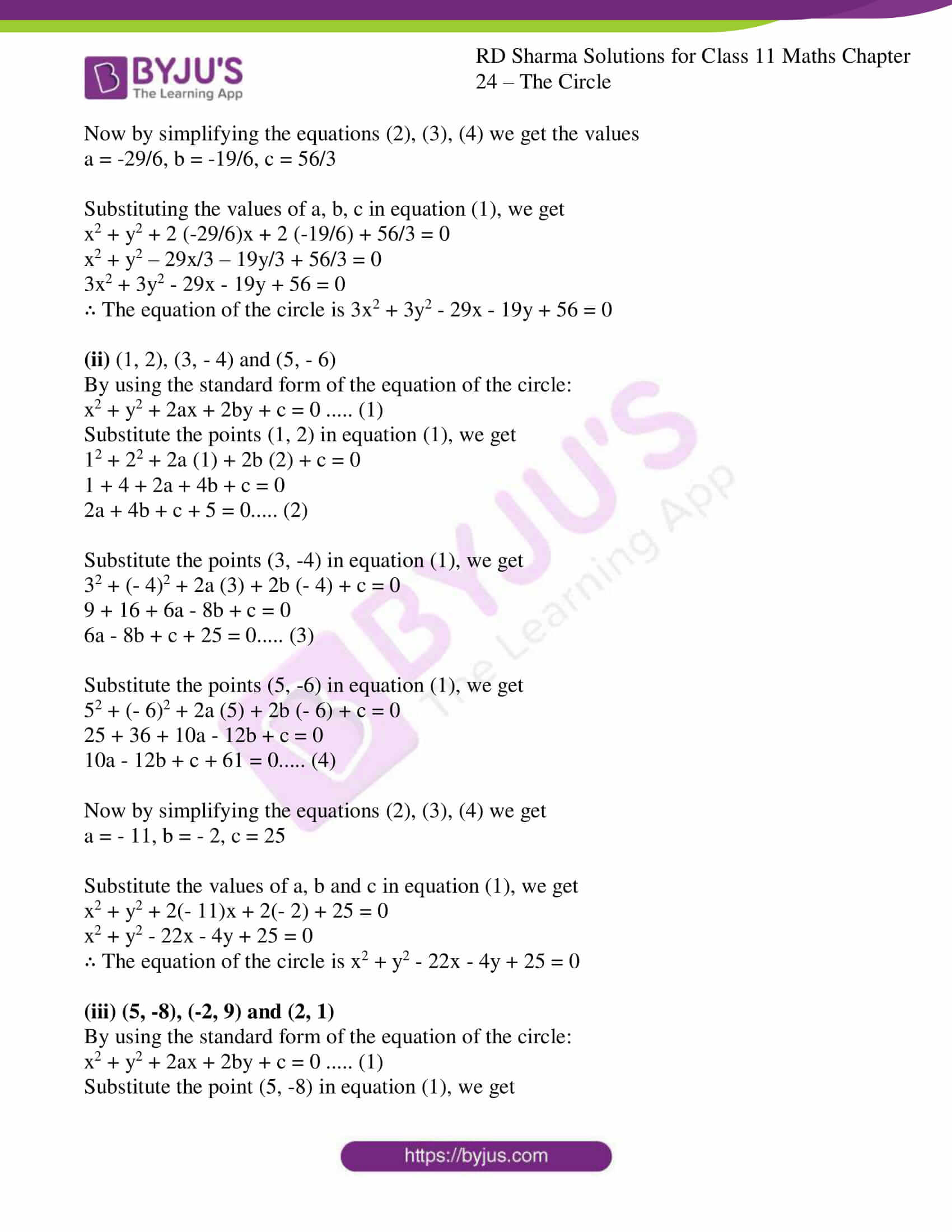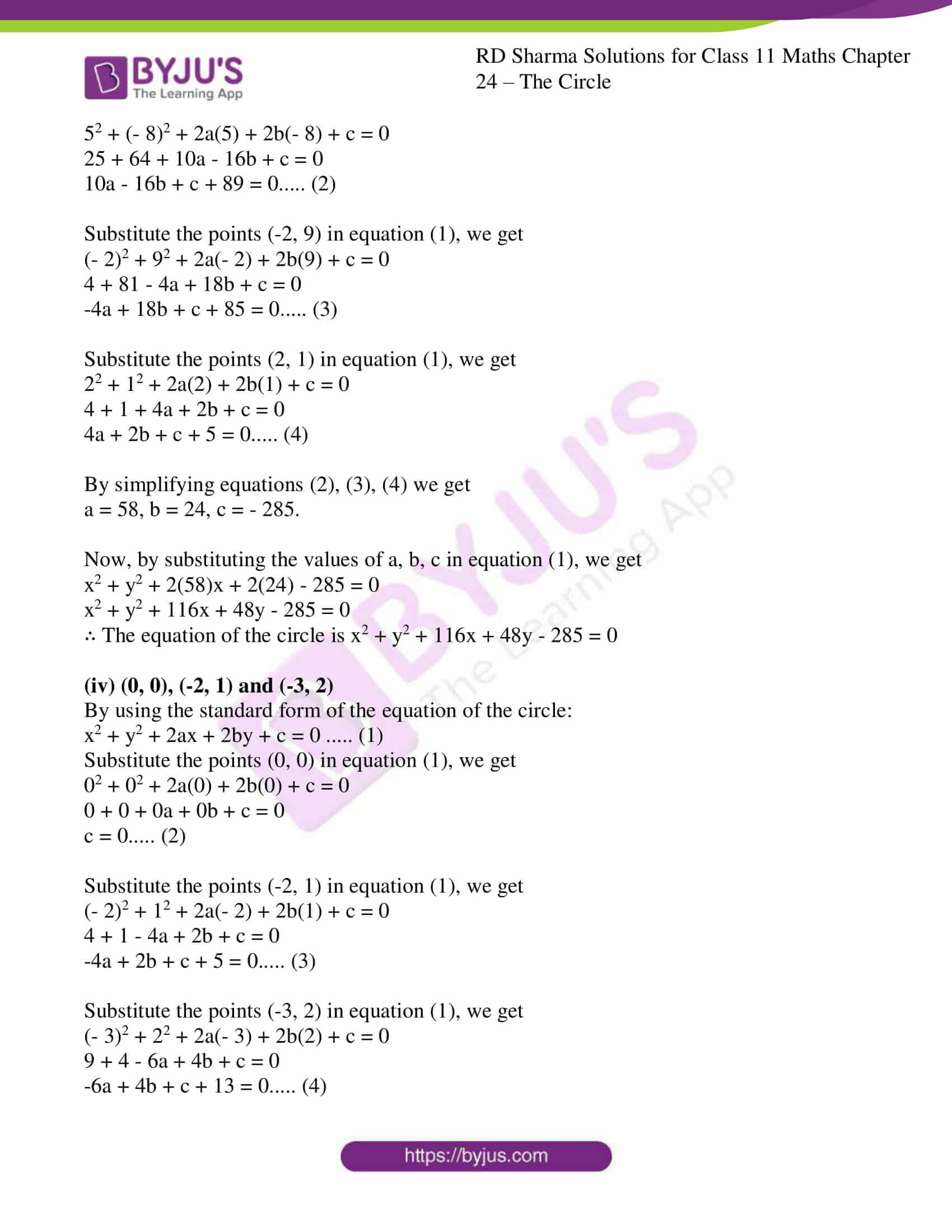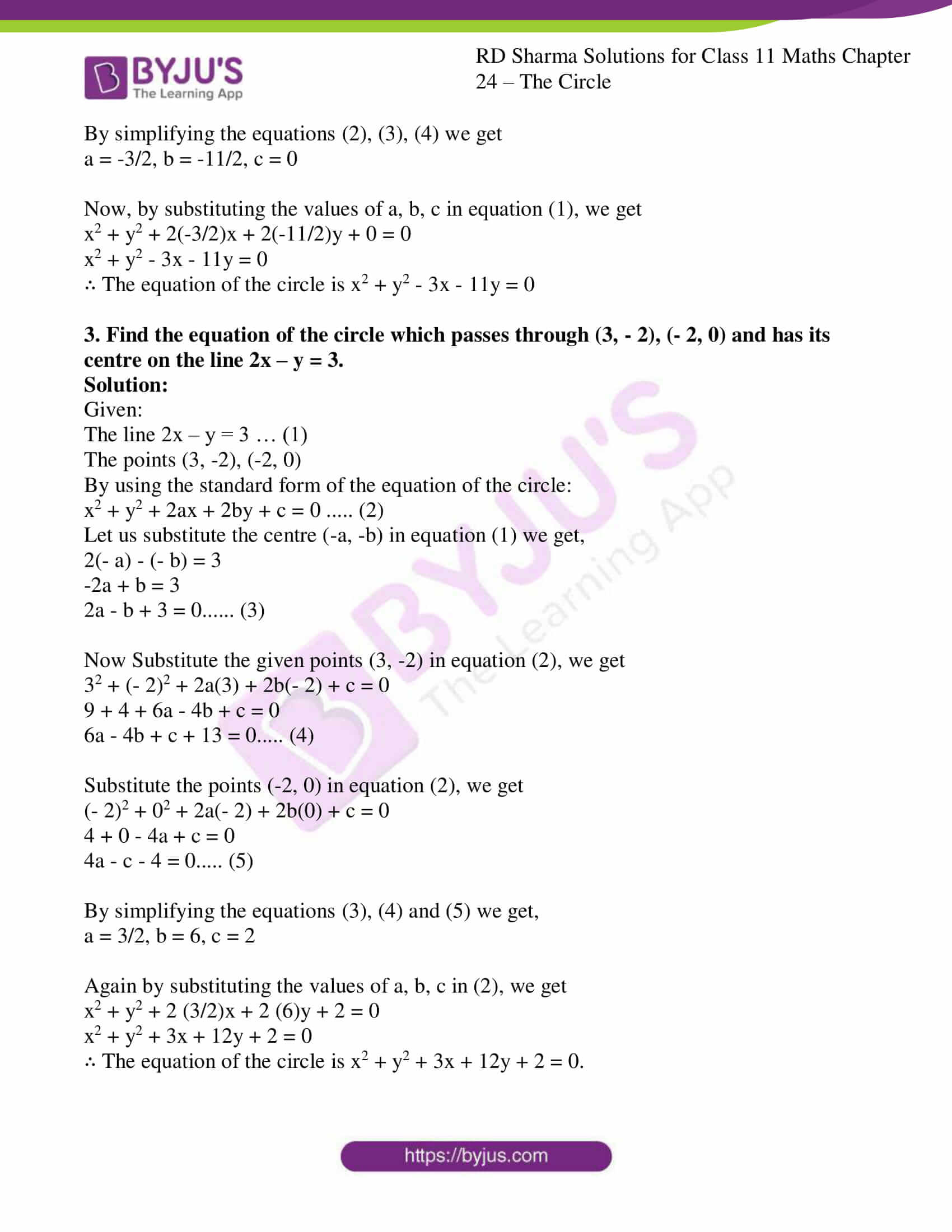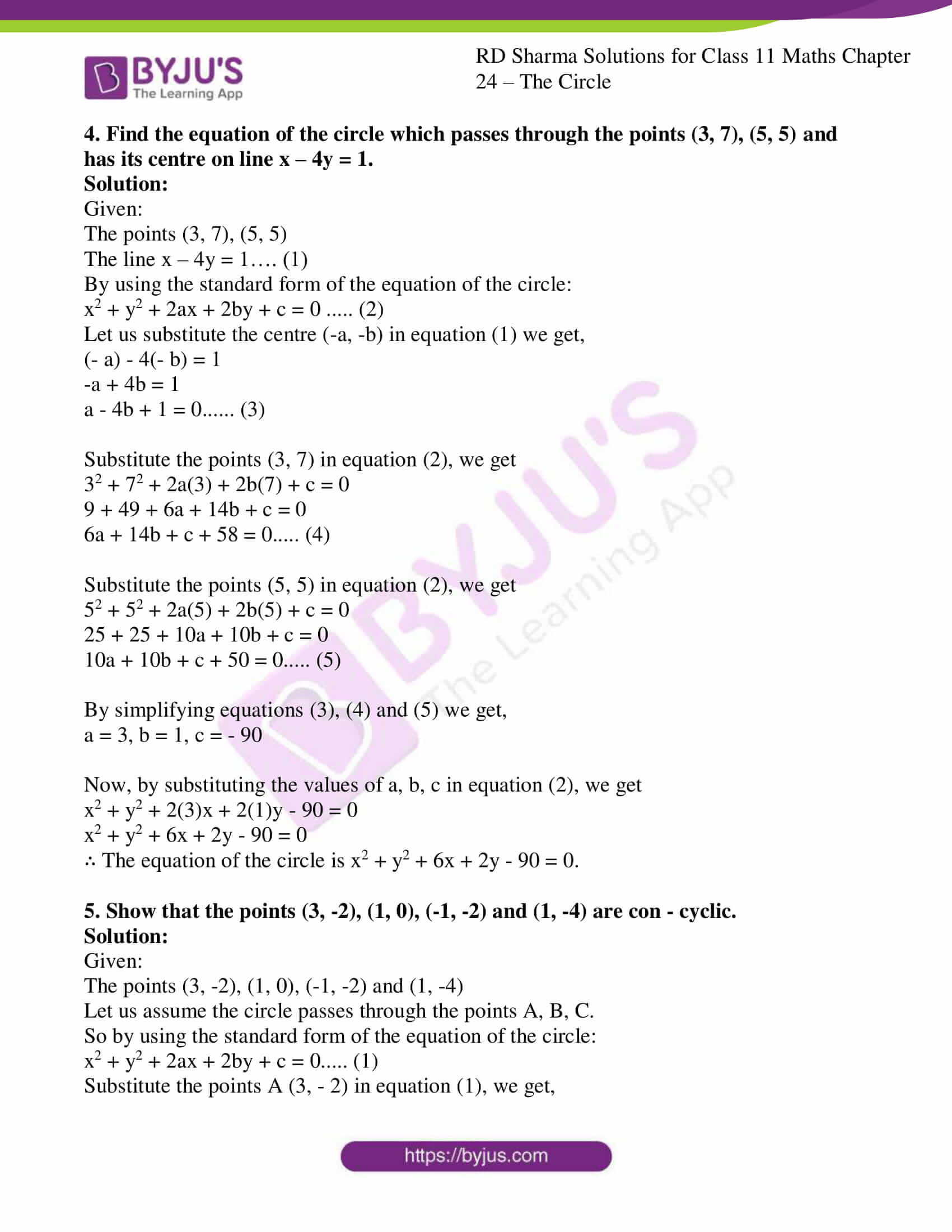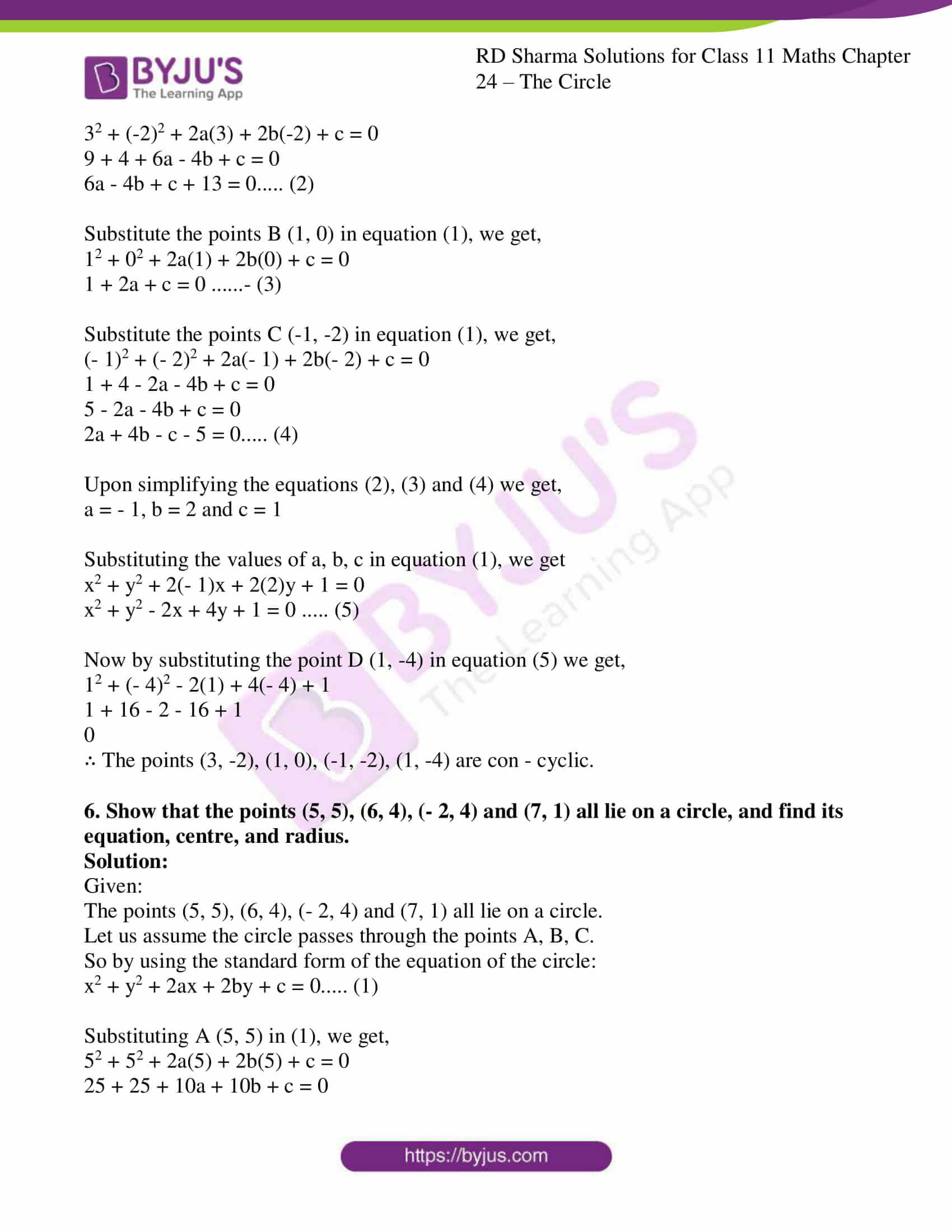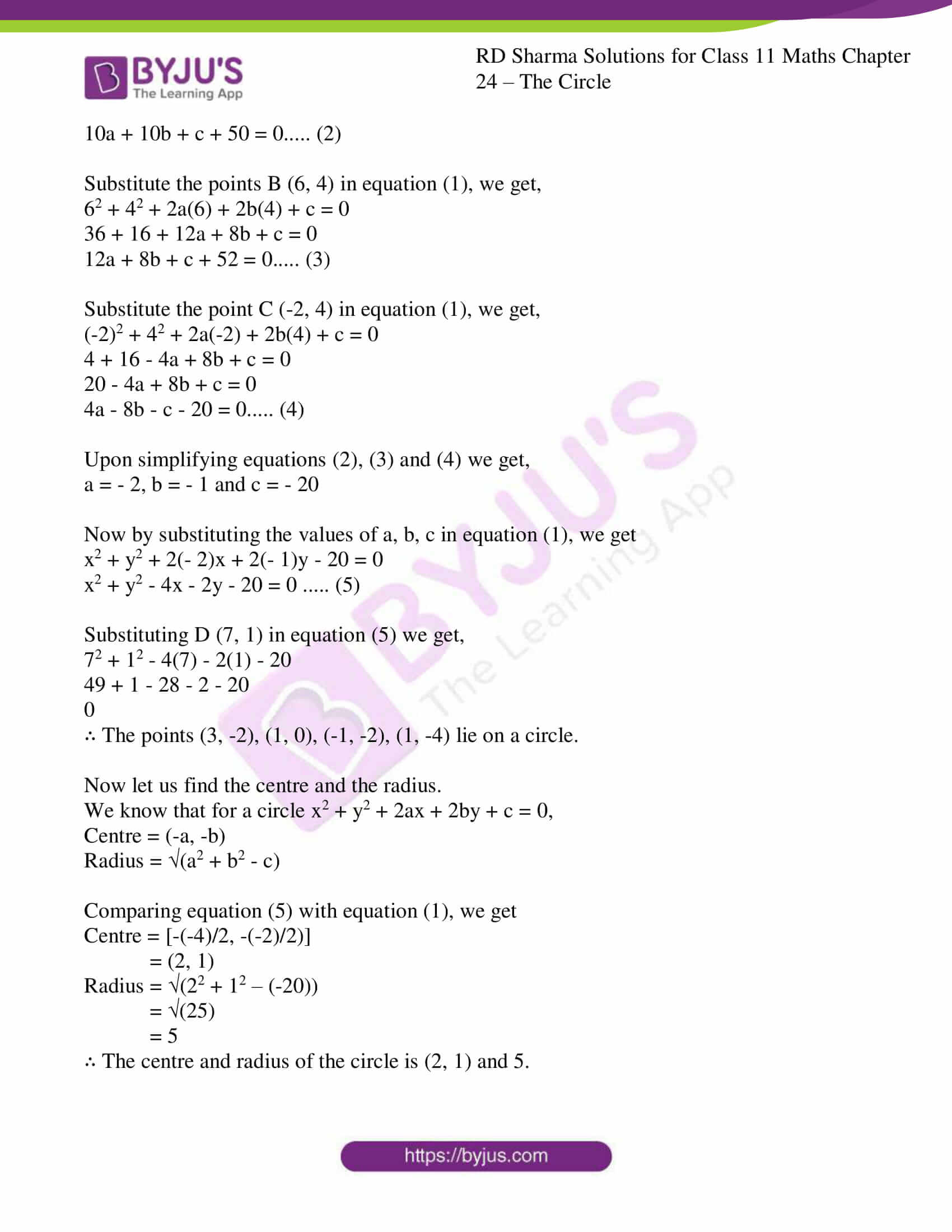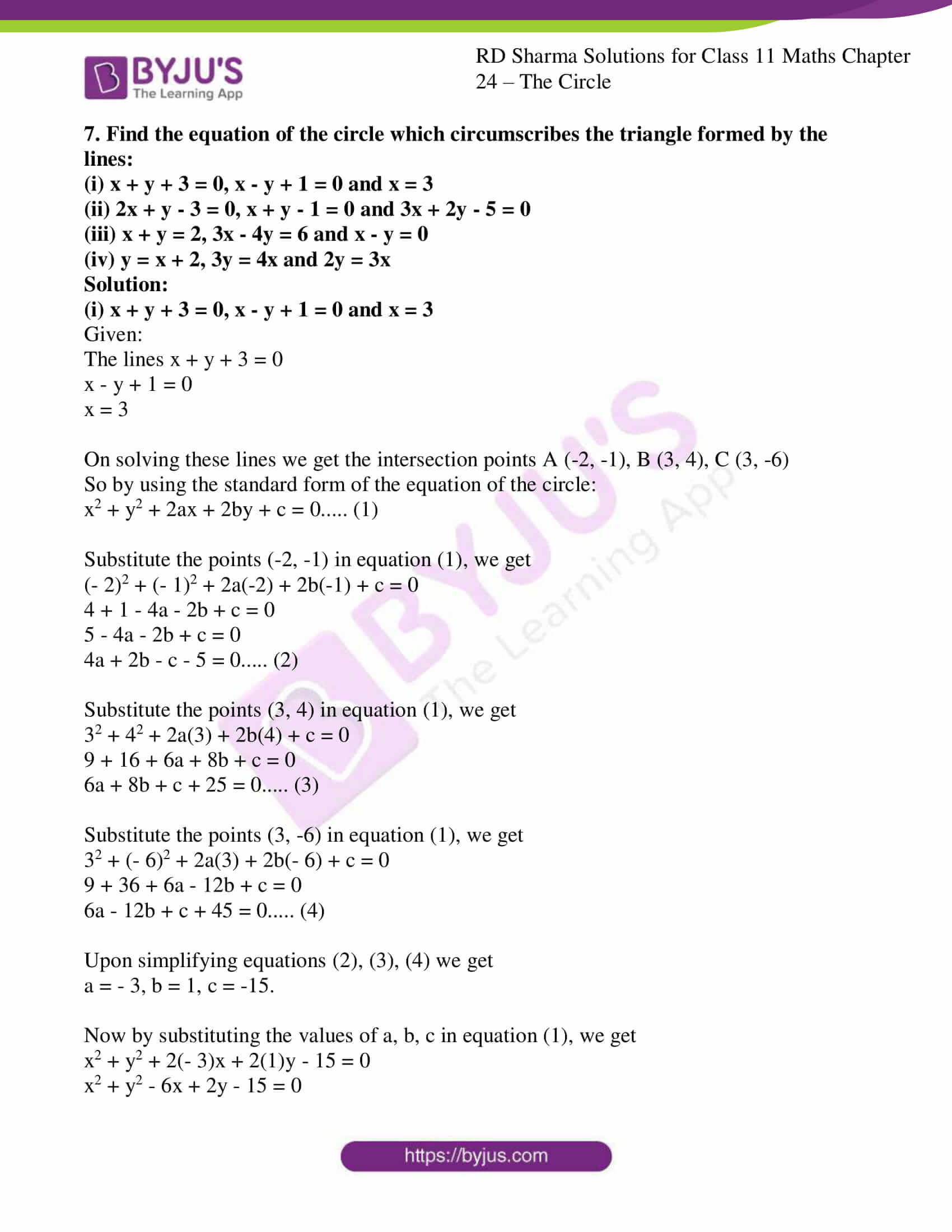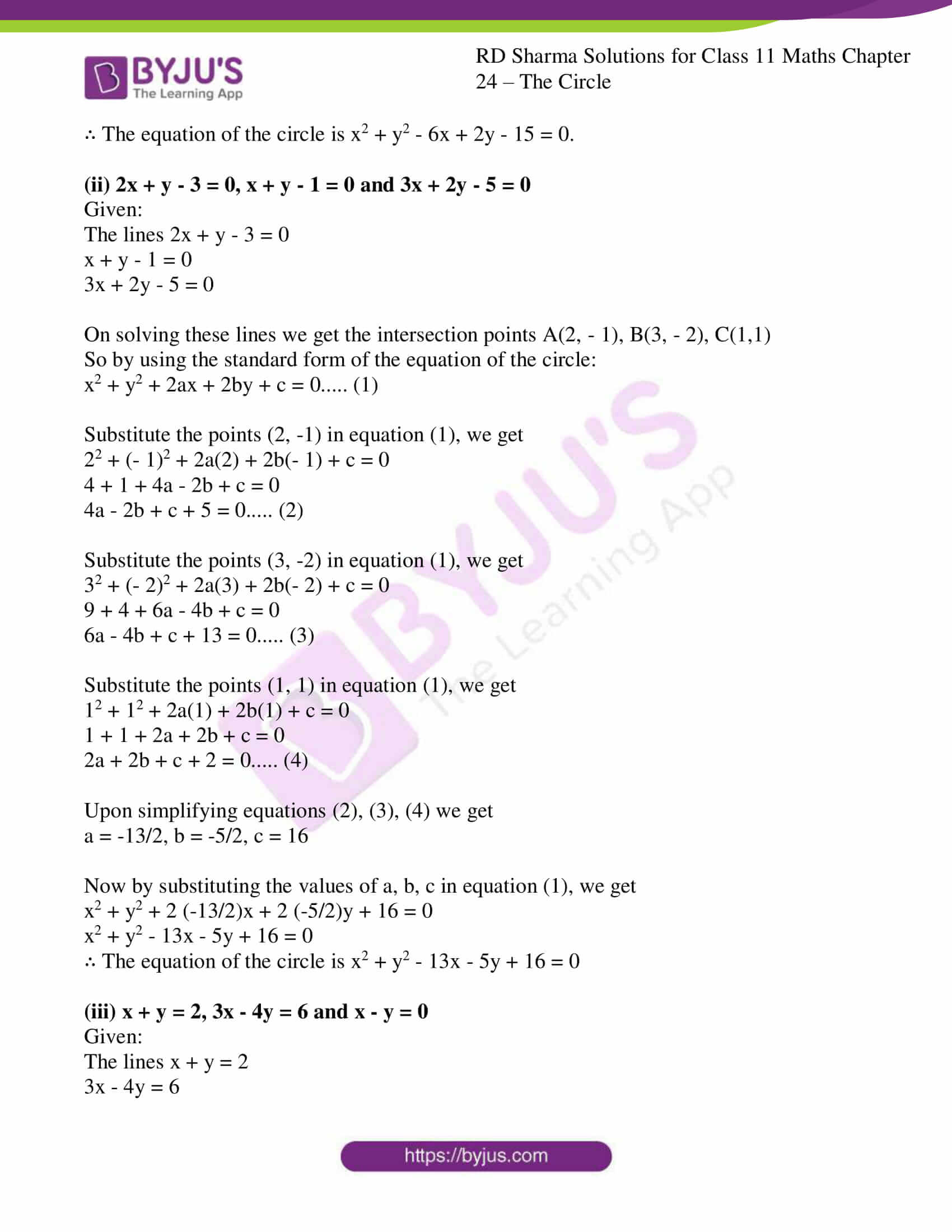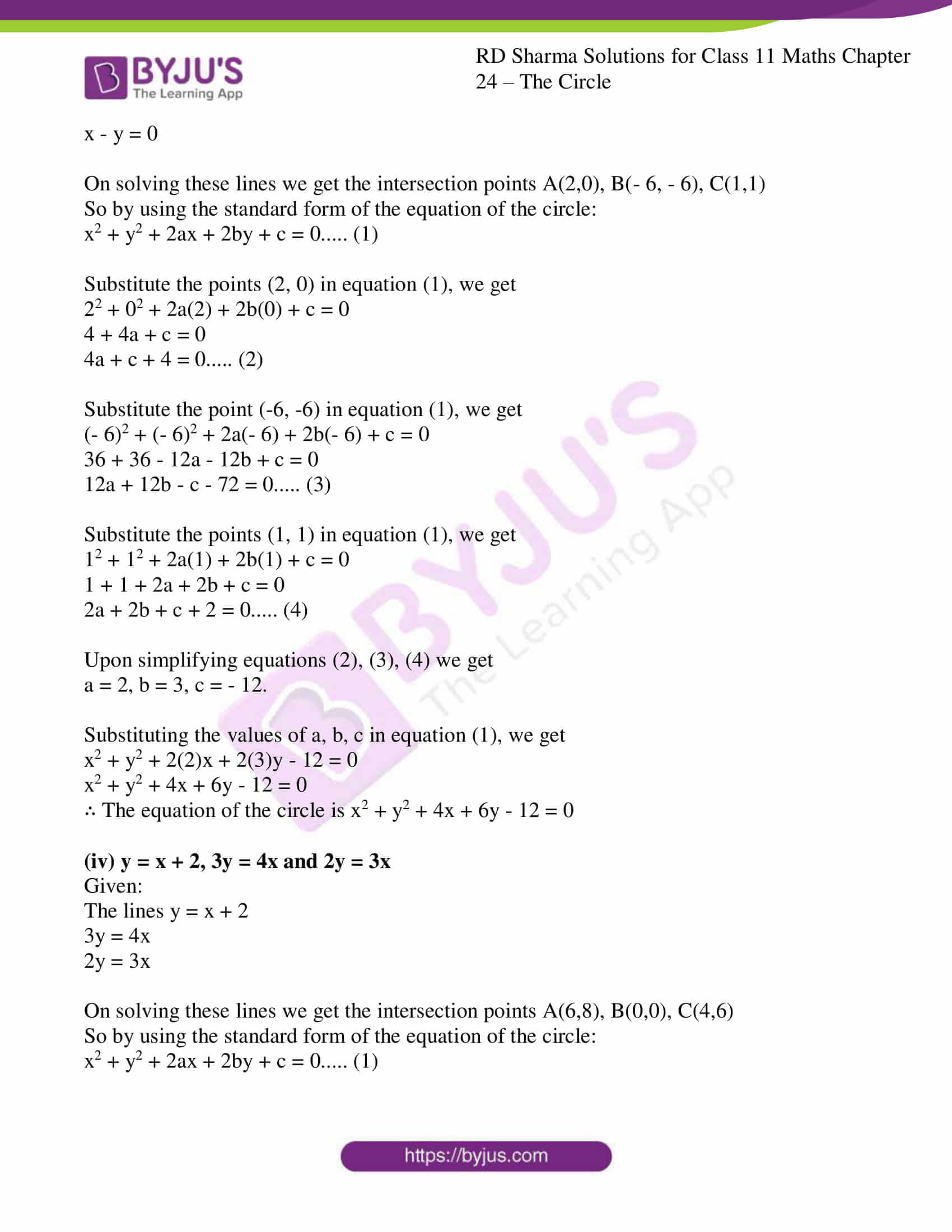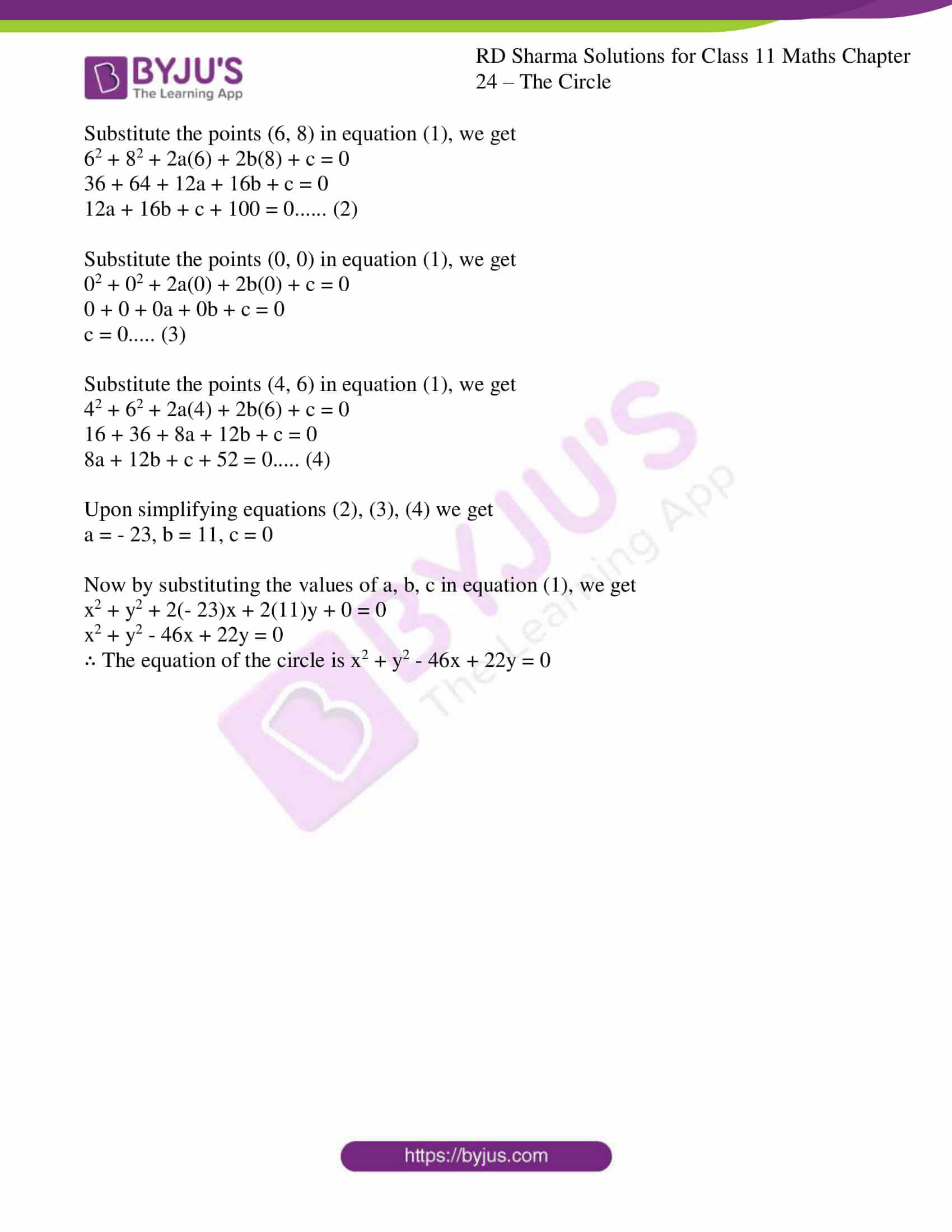### Access answers to RD Sharma Solutions for Class 11 Maths Exercise 24.2 Chapter 24 – The Circle

1. Find the coordinates of the centre radius of each of the following circle:
(i) x2 + y2 + 6x – 8y – 24 = 0

(ii) 2x2 + 2y2 – 3x + 5y = 7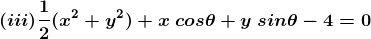(iv) x2 + y2 – ax – by = 0

Solution:

(i) x2 + y2 + 6x – 8y – 24 = 0

Given:

The equation of the circle is x2 + y2 + 6x – 8y – 24 = 0 …… (1)

We know that for a circle x2 + y2 + 2ax + 2by + c = 0 …… (2)

Centre = (-a, -b)

So by comparing equation (1) and (2)

Centre = (-6/2, -(-8)/2)

= (-3, 4)

Radius = (a2 + b2 – c)

= (32 + 42 – (-24))

= (9 + 16 + 24)

= (49)

= 7

∴ The centre of the circle is (-3, 4) and the radius is 7.

(ii) 2x2 + 2y2 – 3x + 5y = 7

Given:

The equation of the circle is 2x2 + 2y2 – 3x + 5y = 7 (divide by 2 we get)

x2 + y2 – 3x/2 + 5y/2 = 7/2

Now, by comparing with the equation x2 + y2 + 2ax + 2by + c = 0

Centre = (-a, -b)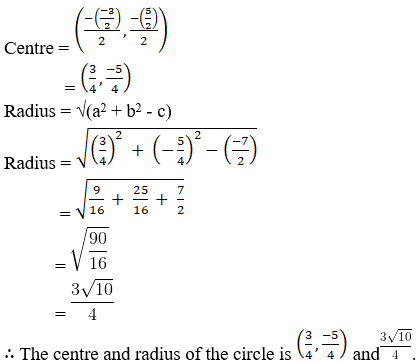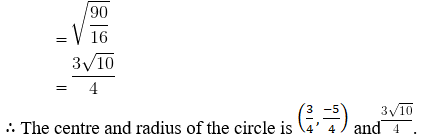Given:

The equation of the circle is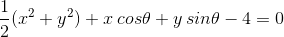(Multiply by 2 we get)

x2 + y2 + 2x cos θ + 2y sin θ – 8 = 0

By comparing with the equation x2 + y2 + 2ax + 2by + c = 0

Centre = (-a, -b)

= [(-2cos θ)/2 , (-2sin θ)/2]

= (-cos θ, -sin θ)

Radius = (a2 + b2 – c)

= √[(-cos θ)2 + (sin θ)2 – (-8)]

= √[cos2 θ + sin2 θ + 8]

= √[1 + 8]

= √

= 3

∴ The centre and radius of the circle is (-cos θ, -sin θ) and 3.

(iv) x2 + y2 – ax – by = 0

Given:

Equation of the circle is x2 + y2 – ax – by = 0

By comparing with the equation x2 + y2 + 2ax + 2by + c = 0

Centre = (-a, -b)

= (-(-a)/2, -(-b)/2)

= (a/2, b/2)

Radius = (a2 + b2 – c)

= √[(a/2)2 + (b/2)2]

= [(a2/4 + b2/4)]

= [(a2 + b2)/4]

= [(a2 + b2)]/2

∴ The centre and radius of the circle is (a/2, b/2) and [(a2 + b2)]/2

2. Find the equation of the circle passing through the points :
(i) (5, 7), (8, 1) and (1, 3)

(ii) (1, 2), (3, – 4) and (5, – 6)

(iii) (5, -8), (-2, 9) and (2, 1)

(iv) (0, 0), (-2, 1) and (-3, 2)

Solution:

(i) (5, 7), (8, 1) and (1, 3)

By using the standard form of the equation of the circle:

x2 + y2 + 2ax + 2by + c = 0 ….. (1)

Firstly let us find the values of a, b and c

Substitute the given point (5, 7) in equation (1), we get

52 + 72 + 2a (5) + 2b (7) + c = 0

25 + 49 + 10a + 14b + c = 0

10a + 14b + c + 74 = 0….. (2)

By substituting the given point (8, 1) in equation (1), we get

82 + 12 + 2a (8) + 2b (1) + c = 0

64 + 1 + 16a + 2b + c = 0

16a + 2b + c + 65 = 0….. (3)

Substituting the point (1, 3) in equation (1), we get

12 + 32 + 2a (1) + 2b (3) + c = 0

1 + 9 + 2a + 6b + c = 0

2a + 6b + c + 10 = 0….. (4)

Now by simplifying the equations (2), (3), (4) we get the values

a = -29/6, b = -19/6, c = 56/3

Substituting the values of a, b, c in equation (1), we get

x2 + y2 + 2 (-29/6)x + 2 (-19/6) + 56/3 = 0

x2 + y2 – 29x/3 – 19y/3 + 56/3 = 0

3x2 + 3y2 – 29x – 19y + 56 = 0

∴ The equation of the circle is 3x2 + 3y2 – 29x – 19y + 56 = 0

(ii) (1, 2), (3, – 4) and (5, – 6)

By using the standard form of the equation of the circle:

x2 + y2 + 2ax + 2by + c = 0 ….. (1)

Substitute the points (1, 2) in equation (1), we get

12 + 22 + 2a (1) + 2b (2) + c = 0

1 + 4 + 2a + 4b + c = 0

2a + 4b + c + 5 = 0….. (2)

Substitute the points (3, -4) in equation (1), we get

32 + (- 4)2 + 2a (3) + 2b (- 4) + c = 0

9 + 16 + 6a – 8b + c = 0

6a – 8b + c + 25 = 0….. (3)

Substitute the points (5, -6) in equation (1), we get

52 + (- 6)2 + 2a (5) + 2b (- 6) + c = 0

25 + 36 + 10a – 12b + c = 0

10a – 12b + c + 61 = 0….. (4)

Now by simplifying the equations (2), (3), (4) we get

a = – 11, b = – 2, c = 25

Substitute the values of a, b and c in equation (1), we get

x2 + y2 + 2(- 11)x + 2(- 2) + 25 = 0

x2 + y2 – 22x – 4y + 25 = 0

∴ The equation of the circle is x2 + y2 – 22x – 4y + 25 = 0

(iii) (5, -8), (-2, 9) and (2, 1)

By using the standard form of the equation of the circle:

x2 + y2 + 2ax + 2by + c = 0 ….. (1)

Substitute the point (5, -8) in equation (1), we get

52 + (- 8)2 + 2a(5) + 2b(- 8) + c = 0

25 + 64 + 10a – 16b + c = 0

10a – 16b + c + 89 = 0….. (2)

Substitute the points (-2, 9) in equation (1), we get

(- 2)2 + 92 + 2a(- 2) + 2b(9) + c = 0

4 + 81 – 4a + 18b + c = 0

-4a + 18b + c + 85 = 0….. (3)

Substitute the points (2, 1) in equation (1), we get

22 + 12 + 2a(2) + 2b(1) + c = 0

4 + 1 + 4a + 2b + c = 0

4a + 2b + c + 5 = 0….. (4)

By simplifying equations (2), (3), (4) we get

a = 58, b = 24, c = – 285.

Now, by substituting the values of a, b, c in equation (1), we get

x2 + y2 + 2(58)x + 2(24) – 285 = 0

x2 + y2 + 116x + 48y – 285 = 0

∴ The equation of the circle is x2 + y2 + 116x + 48y – 285 = 0

(iv) (0, 0), (-2, 1) and (-3, 2)

By using the standard form of the equation of the circle:

x2 + y2 + 2ax + 2by + c = 0 ….. (1)

Substitute the points (0, 0) in equation (1), we get

02 + 02 + 2a(0) + 2b(0) + c = 0

0 + 0 + 0a + 0b + c = 0

c = 0….. (2)

Substitute the points (-2, 1) in equation (1), we get

(- 2)2 + 12 + 2a(- 2) + 2b(1) + c = 0

4 + 1 – 4a + 2b + c = 0

-4a + 2b + c + 5 = 0….. (3)

Substitute the points (-3, 2) in equation (1), we get

(- 3)2 + 22 + 2a(- 3) + 2b(2) + c = 0

9 + 4 – 6a + 4b + c = 0

-6a + 4b + c + 13 = 0….. (4)

By simplifying the equations (2), (3), (4) we get

a = -3/2, b = -11/2, c = 0

Now, by substituting the values of a, b, c in equation (1), we get

x2 + y2 + 2(-3/2)x + 2(-11/2)y + 0 = 0

x2 + y2 – 3x – 11y = 0

∴ The equation of the circle is x2 + y2 – 3x – 11y = 0

3. Find the equation of the circle which passes through (3, – 2), (- 2, 0) and has its centre on the line 2x – y = 3.

Solution:

Given:

The line 2x – y = 3 … (1)

The points (3, -2), (-2, 0)

By using the standard form of the equation of the circle:

x2 + y2 + 2ax + 2by + c = 0 ….. (2)

Let us substitute the centre (-a, -b) in equation (1) we get,

2(- a) – (- b) = 3

-2a + b = 3

2a – b + 3 = 0…… (3)

Now Substitute the given points (3, -2) in equation (2), we get

32 + (- 2)2 + 2a(3) + 2b(- 2) + c = 0

9 + 4 + 6a – 4b + c = 0

6a – 4b + c + 13 = 0….. (4)

Substitute the points (-2, 0) in equation (2), we get

(- 2)2 + 02 + 2a(- 2) + 2b(0) + c = 0

4 + 0 – 4a + c = 0

4a – c – 4 = 0….. (5)

By simplifying the equations (3), (4) and (5) we get,

a = 3/2, b = 6, c = 2

Again by substituting the values of a, b, c in (2), we get

x2 + y2 + 2 (3/2)x + 2 (6)y + 2 = 0

x2 + y2 + 3x + 12y + 2 = 0

∴ The equation of the circle is x2 + y2 + 3x + 12y + 2 = 0.

4. Find the equation of the circle which passes through the points (3, 7), (5, 5) and has its centre on line x – 4y = 1.

Solution:

Given:

The points (3, 7), (5, 5)

The line x – 4y = 1…. (1)

By using the standard form of the equation of the circle:

x2 + y2 + 2ax + 2by + c = 0 ….. (2)

Let us substitute the centre (-a, -b) in equation (1) we get,

(- a) – 4(- b) = 1

-a + 4b = 1

a – 4b + 1 = 0…… (3)

Substitute the points (3, 7) in equation (2), we get

32 + 72 + 2a(3) + 2b(7) + c = 0

9 + 49 + 6a + 14b + c = 0

6a + 14b + c + 58 = 0….. (4)

Substitute the points (5, 5) in equation (2), we get

52 + 52 + 2a(5) + 2b(5) + c = 0

25 + 25 + 10a + 10b + c = 0

10a + 10b + c + 50 = 0….. (5)

By simplifying equations (3), (4) and (5) we get,

a = 3, b = 1, c = – 90

Now, by substituting the values of a, b, c in equation (2), we get

x2 + y2 + 2(3)x + 2(1)y – 90 = 0

x2 + y2 + 6x + 2y – 90 = 0

∴ The equation of the circle is x2 + y2 + 6x + 2y – 90 = 0.

5. Show that the points (3, -2), (1, 0), (-1, -2) and (1, -4) are con – cyclic.

Solution:

Given:

The points (3, -2), (1, 0), (-1, -2) and (1, -4)

Let us assume the circle passes through the points A, B, C.

So by using the standard form of the equation of the circle:

x2 + y2 + 2ax + 2by + c = 0….. (1)

Substitute the points A (3, – 2) in equation (1), we get,

32 + (-2)2 + 2a(3) + 2b(-2) + c = 0

9 + 4 + 6a – 4b + c = 0

6a – 4b + c + 13 = 0….. (2)

Substitute the points B (1, 0) in equation (1), we get,

12 + 02 + 2a(1) + 2b(0) + c = 0

1 + 2a + c = 0 ……- (3)

Substitute the points C (-1, -2) in equation (1), we get,

(- 1)2 + (- 2)2 + 2a(- 1) + 2b(- 2) + c = 0

1 + 4 – 2a – 4b + c = 0

5 – 2a – 4b + c = 0

2a + 4b – c – 5 = 0….. (4)

Upon simplifying the equations (2), (3) and (4) we get,

a = – 1, b = 2 and c = 1

Substituting the values of a, b, c in equation (1), we get

x2 + y2 + 2(- 1)x + 2(2)y + 1 = 0

x2 + y2 – 2x + 4y + 1 = 0 ….. (5)

Now by substituting the point D (1, -4) in equation (5) we get,

12 + (- 4)2 – 2(1) + 4(- 4) + 1

1 + 16 – 2 – 16 + 1

0

∴ The points (3, -2), (1, 0), (-1, -2), (1, -4) are con – cyclic.

6. Show that the points (5, 5), (6, 4), (- 2, 4) and (7, 1) all lie on a circle, and find its equation, centre, and radius.

Solution:

Given:

The points (5, 5), (6, 4), (- 2, 4) and (7, 1) all lie on a circle.

Let us assume the circle passes through the points A, B, C.

So by using the standard form of the equation of the circle:

x2 + y2 + 2ax + 2by + c = 0….. (1)

Substituting A (5, 5) in (1), we get,

52 + 52 + 2a(5) + 2b(5) + c = 0

25 + 25 + 10a + 10b + c = 0

10a + 10b + c + 50 = 0….. (2)

Substitute the points B (6, 4) in equation (1), we get,

62 + 42 + 2a(6) + 2b(4) + c = 0

36 + 16 + 12a + 8b + c = 0

12a + 8b + c + 52 = 0….. (3)

Substitute the point C (-2, 4) in equation (1), we get,

(-2)2 + 42 + 2a(-2) + 2b(4) + c = 0

4 + 16 – 4a + 8b + c = 0

20 – 4a + 8b + c = 0

4a – 8b – c – 20 = 0….. (4)

Upon simplifying equations (2), (3) and (4) we get,

a = – 2, b = – 1 and c = – 20

Now by substituting the values of a, b, c in equation (1), we get

x2 + y2 + 2(- 2)x + 2(- 1)y – 20 = 0

x2 + y2 – 4x – 2y – 20 = 0 ….. (5)

Substituting D (7, 1) in equation (5) we get,

72 + 12 – 4(7) – 2(1) – 20

49 + 1 – 28 – 2 – 20

0

∴ The points (3, -2), (1, 0), (-1, -2), (1, -4) lie on a circle.

Now let us find the centre and the radius.

We know that for a circle x2 + y2 + 2ax + 2by + c = 0,

Centre = (-a, -b)

Radius = (a2 + b2 – c)

Comparing equation (5) with equation (1), we get

Centre = [-(-4)/2, -(-2)/2)]

= (2, 1)

Radius = (22 + 12 – (-20))

= (25)

= 5

∴ The centre and radius of the circle is (2, 1) and 5.

7. Find the equation of the circle which circumscribes the triangle formed by the lines:
(i) x + y + 3 = 0, x – y + 1 = 0 and x = 3

(ii) 2x + y – 3 = 0, x + y – 1 = 0 and 3x + 2y – 5 = 0

(iii) x + y = 2, 3x – 4y = 6 and x – y = 0

(iv) y = x + 2, 3y = 4x and 2y = 3x

Solution:

(i) x + y + 3 = 0, x – y + 1 = 0 and x = 3

Given:

The lines x + y + 3 = 0

x – y + 1 = 0

x = 3

On solving these lines we get the intersection points A (-2, -1), B (3, 4), C (3, -6)

So by using the standard form of the equation of the circle:

x2 + y2 + 2ax + 2by + c = 0….. (1)

Substitute the points (-2, -1) in equation (1), we get

(- 2)2 + (- 1)2 + 2a(-2) + 2b(-1) + c = 0

4 + 1 – 4a – 2b + c = 0

5 – 4a – 2b + c = 0

4a + 2b – c – 5 = 0….. (2)

Substitute the points (3, 4) in equation (1), we get

32 + 42 + 2a(3) + 2b(4) + c = 0

9 + 16 + 6a + 8b + c = 0

6a + 8b + c + 25 = 0….. (3)

Substitute the points (3, -6) in equation (1), we get

32 + (- 6)2 + 2a(3) + 2b(- 6) + c = 0

9 + 36 + 6a – 12b + c = 0

6a – 12b + c + 45 = 0….. (4)

Upon simplifying equations (2), (3), (4) we get

a = – 3, b = 1, c = -15.

Now by substituting the values of a, b, c in equation (1), we get

x2 + y2 + 2(- 3)x + 2(1)y – 15 = 0

x2 + y2 – 6x + 2y – 15 = 0

∴ The equation of the circle is x2 + y2 – 6x + 2y – 15 = 0.

(ii) 2x + y – 3 = 0, x + y – 1 = 0 and 3x + 2y – 5 = 0

Given:

The lines 2x + y – 3 = 0

x + y – 1 = 0

3x + 2y – 5 = 0

On solving these lines we get the intersection points A(2, – 1), B(3, – 2), C(1,1)

So by using the standard form of the equation of the circle:

x2 + y2 + 2ax + 2by + c = 0….. (1)

Substitute the points (2, -1) in equation (1), we get

22 + (- 1)2 + 2a(2) + 2b(- 1) + c = 0

4 + 1 + 4a – 2b + c = 0

4a – 2b + c + 5 = 0….. (2)

Substitute the points (3, -2) in equation (1), we get

32 + (- 2)2 + 2a(3) + 2b(- 2) + c = 0

9 + 4 + 6a – 4b + c = 0

6a – 4b + c + 13 = 0….. (3)

Substitute the points (1, 1) in equation (1), we get

12 + 12 + 2a(1) + 2b(1) + c = 0

1 + 1 + 2a + 2b + c = 0

2a + 2b + c + 2 = 0….. (4)

Upon simplifying equations (2), (3), (4) we get

a = -13/2, b = -5/2, c = 16

Now by substituting the values of a, b, c in equation (1), we get

x2 + y2 + 2 (-13/2)x + 2 (-5/2)y + 16 = 0

x2 + y2 – 13x – 5y + 16 = 0

∴ The equation of the circle is x2 + y2 – 13x – 5y + 16 = 0

(iii) x + y = 2, 3x – 4y = 6 and x – y = 0

Given:

The lines x + y = 2

3x – 4y = 6

x – y = 0

On solving these lines we get the intersection points A(2,0), B(- 6, – 6), C(1,1)

So by using the standard form of the equation of the circle:

x2 + y2 + 2ax + 2by + c = 0….. (1)

Substitute the points (2, 0) in equation (1), we get

22 + 02 + 2a(2) + 2b(0) + c = 0

4 + 4a + c = 0

4a + c + 4 = 0….. (2)

Substitute the point (-6, -6) in equation (1), we get

(- 6)2 + (- 6)2 + 2a(- 6) + 2b(- 6) + c = 0

36 + 36 – 12a – 12b + c = 0

12a + 12b – c – 72 = 0….. (3)

Substitute the points (1, 1) in equation (1), we get

12 + 12 + 2a(1) + 2b(1) + c = 0

1 + 1 + 2a + 2b + c = 0

2a + 2b + c + 2 = 0….. (4)

Upon simplifying equations (2), (3), (4) we get

a = 2, b = 3, c = – 12.

Substituting the values of a, b, c in equation (1), we get

x2 + y2 + 2(2)x + 2(3)y – 12 = 0

x2 + y2 + 4x + 6y – 12 = 0

∴ The equation of the circle is x2 + y2 + 4x + 6y – 12 = 0

(iv) y = x + 2, 3y = 4x and 2y = 3x

Given:

The lines y = x + 2

3y = 4x

2y = 3x

On solving these lines we get the intersection points A(6,8), B(0,0), C(4,6)

So by using the standard form of the equation of the circle:

x2 + y2 + 2ax + 2by + c = 0….. (1)

Substitute the points (6, 8) in equation (1), we get

62 + 82 + 2a(6) + 2b(8) + c = 0

36 + 64 + 12a + 16b + c = 0

12a + 16b + c + 100 = 0…… (2)

Substitute the points (0, 0) in equation (1), we get

02 + 02 + 2a(0) + 2b(0) + c = 0

0 + 0 + 0a + 0b + c = 0

c = 0….. (3)

Substitute the points (4, 6) in equation (1), we get

42 + 62 + 2a(4) + 2b(6) + c = 0

16 + 36 + 8a + 12b + c = 0

8a + 12b + c + 52 = 0….. (4)

Upon simplifying equations (2), (3), (4) we get

a = – 23, b = 11, c = 0

Now by substituting the values of a, b, c in equation (1), we get

x2 + y2 + 2(- 23)x + 2(11)y + 0 = 0

x2 + y2 – 46x + 22y = 0

∴ The equation of the circle is x2 + y2 – 46x + 22y = 0